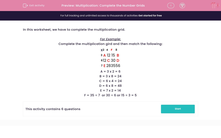# Complete Number Grids Using Multiplication Facts

In this worksheet, students will use both multiplication and division in order to complete a grid of numbers.This content is premium and exclusive to EdPlace subscribers.Key stage:  KS 2

Curriculum topic:   Maths and Numerical Reasoning

Curriculum subtopic:   Mixed Problems

Difficulty level:#### Worksheet Overview

In this activity, we have to complete the multiplication grid.

Example

Complete the multiplication grid and then match the following:

x

2 4 F 8
3 A 12 15 B
6 12 C 30 D
7 E 28 35 56

A = 3 x 2 = 6

B = 3 x 8 = 24

C = 6 x 4 = 24

D = 6 x 8 = 48

E = 7 x 2 = 14

F = 35 ÷ 7  or 30 ÷ 6 or 15 ÷ 3  They all give the same answer of 5.

Are you ready to get started?

### What is EdPlace?

We're your National Curriculum aligned online education content provider helping each child succeed in English, maths and science from year 1 to GCSE. With an EdPlace account you’ll be able to track and measure progress, helping each child achieve their best. We build confidence and attainment by personalising each child’s learning at a level that suits them.

Get started